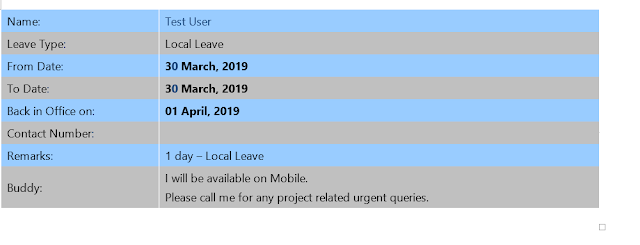## Monday, March 30, 2020

This blogs explains how you can read the email contents and add to an excel file in SharePoint online using power automate (flow)

Below are the actions involve in this.

•  Add a "Compose" action for each value you want to extract from your email, Inputs set to following formula (Sample email below)
•To grab any of those fields in Flow you use a formula like this (example grabs the email)
trim(
substring(
body('Html_to_text'),
indexOf(
body('Html_to_text'),
'Name:'),
7),
sub(
indexOf(
body('Html_to_text'),
'Leave Type:'),
indexOf(
body('Html_to_text'),
'Name:'),
7)
)
)
)

The breakdow of above formula is given below.
The subsstring() function has 3 inputs, 1. the source string, 2. the start index, which is the number of characters into the string to start looking and 3. the length.

The objective therefore, is to derive 2. and 3. so we can grab the relevant text no matter how long is it.

So firstly we need to know how many characters into the source text to start. This is relatively easy:
indexOf(
body('Html_to_text'),
'Name:'),
7)
he indexOf() function finds the number of characters into a text string that a string occurs. Here we are calculating the indexOf() the string "Name: " it doesn't matter where in the original message body this occurs, indexOf() will find it and return a number.

Now we don't actually want the string "Name: " in the output of this expression so we now add() a number to the output of indexOf() to get the number of characters into the message body the end of "Name: " occurs. We don't calculate this dynamically; it's 7, including the space.

So by using indexOf() to find the place in the source string where "Name: " exists, then adding that number of characters to it, we get the point we want to start reading the email address.

Next we have to calculate the length of the string, for the 3rd parameter of substring():
sub(
indexOf(
body('Html_to_text'),
'Leave Type:'),
indexOf(
body('Html_to_text'),
'Name:'),
7)
)
To do this we use the sub() function to subtract the number we just calculated from the indexOf() the place we want to stop looking. Thus a lot (the second parameter of sub()) of the expression above is similar to the one before - calculating the number of characters into the source string that our relevant text starts.

The first parameter of sub() is the place where our relevant text ends - which in this case is the start of the string "Leave Type: ". We don't need to do anything complex with this because it's the start of the string we want the index of, not the end.

So we now have our 3 parameters for substring(), the source text, which is body('Html_to_text') in this example, the number of characters into that where we find the start of our substring and lastly length, which is calculated by subtracting the former from the number of characters into the source text where we want to stop looking.

Finally, in my example I've wrapped trim() around the whole thing to eliminate any trailing whitespace.

Send an email to the mailbox and you will see the selected rows added to your excel.